t molloy

•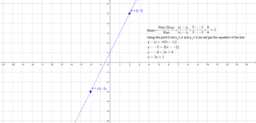investigating the equation of a line formula

Activity

t molloy

•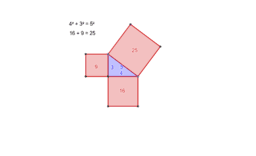Pythagoras' Theorem

Activity

t molloy

•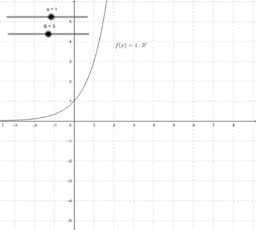Copy of Exponential Function Investigation.

Activity

t molloy

•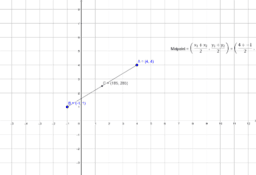investigating the midpoint formula

Activity

t molloy

•Investigation of circumference

Activity

t molloy

•coordinates examples

Activity

t molloy

•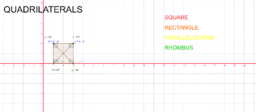Activity

t molloy

•corresponding angles

Activity

t molloy

•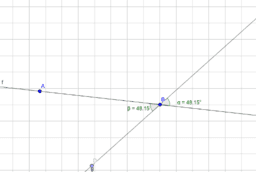opposi

Activity

t molloy

•Practice Addition and Subtraction of Algebraic Frac

Activity

t molloy

•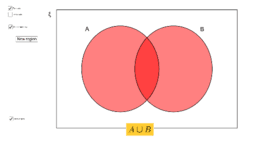Venn diagram shading with 2 and 3 sets

Activity

t molloy

•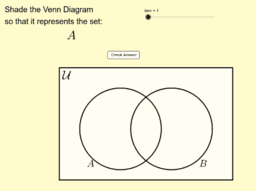Activity

t molloy

•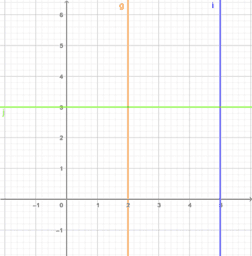vertical and horizontal lines

Activity

t molloy

•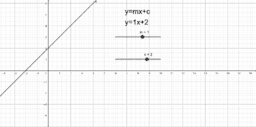Activity

t molloy